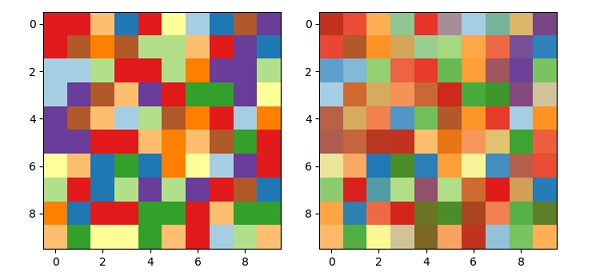# How to extract a subset of a colormap as a new colormap in Matplotlib?

To extract a subset of a colormap as a new colormap, we can take the following steps −

• Create a random array with 10×10 shape.

• Add a subplot to the current figure, where nrows=1, ncols=2 and index=1.

• Initialize using get_cmap so that scatter knows.

• Using imshow() method with colormap, display the data as an image, i.e., on a 2D regular raster, with data and colormap (Steps 1 and 3).

• Add a subplot to the current figure, where nrows=1, ncols=2 and index=2.

• Extract a subset of the colormap from the existing colormap (From step 3).

• Using imshow() method with colormap, display the data as an image, i.e., on a 2D regular raster, with data and colormap (Steps 1 and 6).

• To display the figure, use the show() method.

## Example

import numpy as np
from matplotlib import pyplot as plt, colors
import matplotlib
plt.rcParams["figure.figsize"] = [7.50, 3.50]
plt.rcParams["figure.autolayout"] = True
data = np.random.rand(10, 10)
plt.subplot(121)
cmap = matplotlib.cm.get_cmap('Paired_r', 10)
plt.imshow(data, cmap=cmap)
plt.subplot(122)
new_cmap = colors.LinearSegmentedColormap.from_list(cmap.name, cmap.colors)
plt.imshow(data, cmap=new_cmap)
plt.show()

## Output